Ex 9.4

Chapter 9 Class 12 Differential Equations
Serial order wise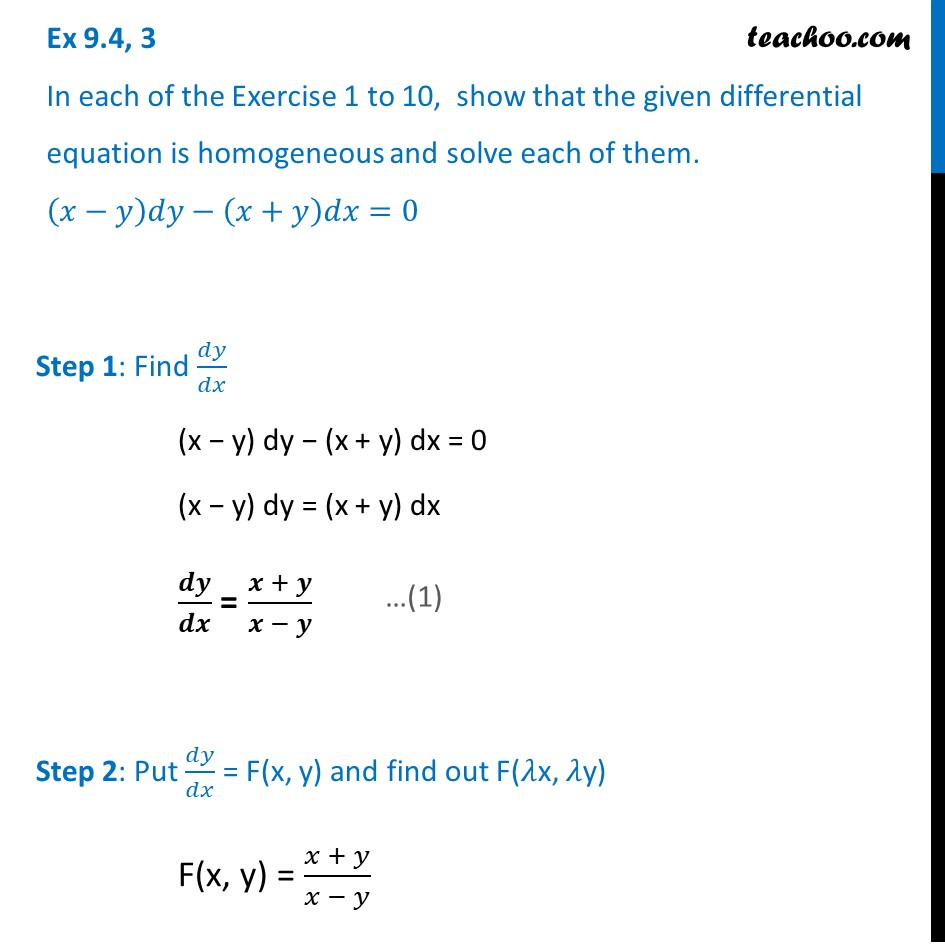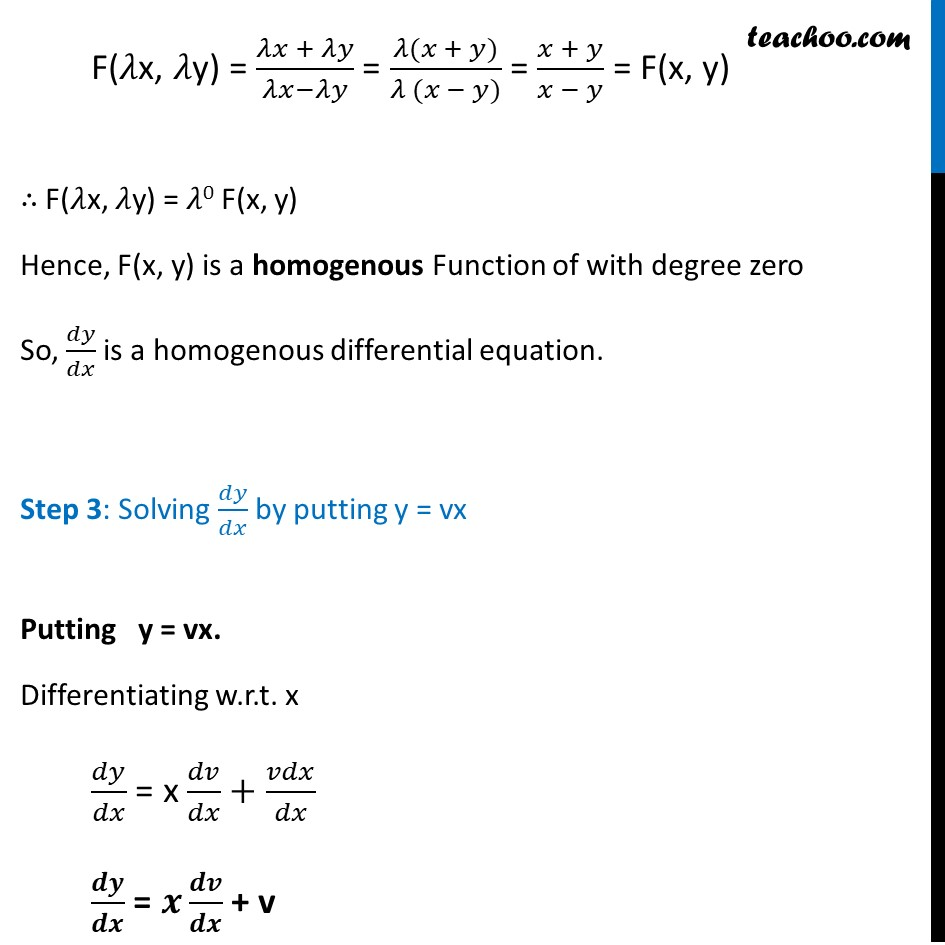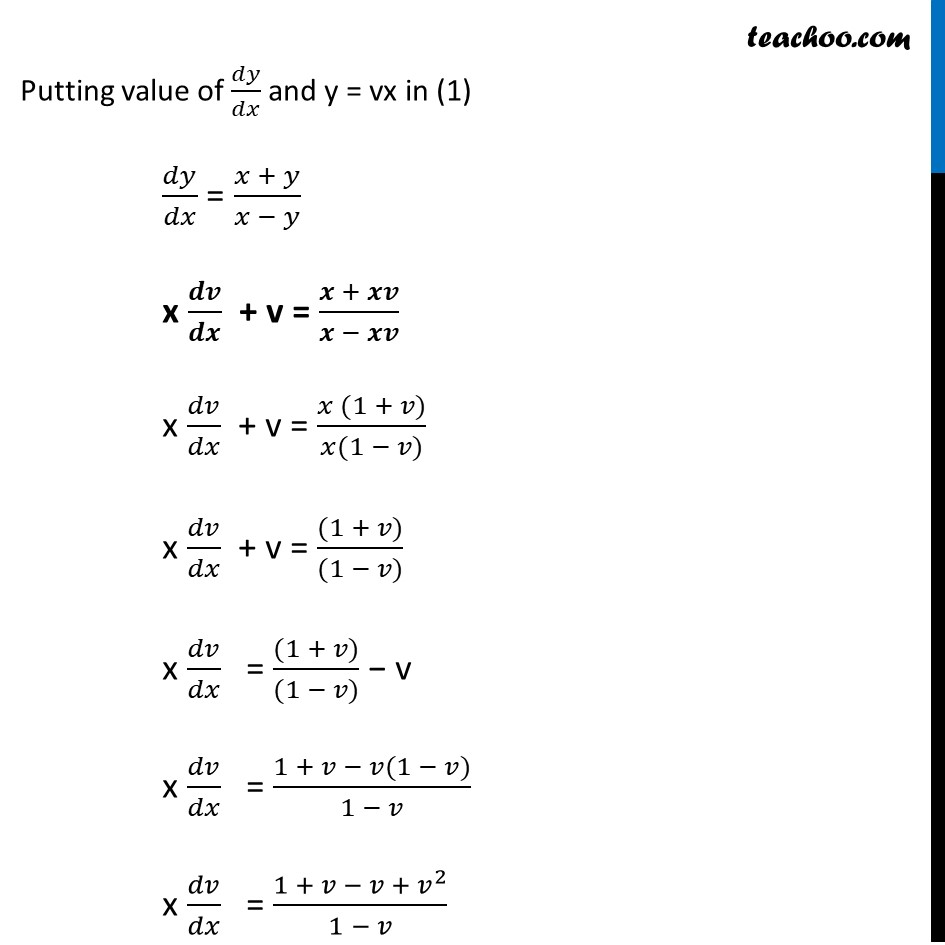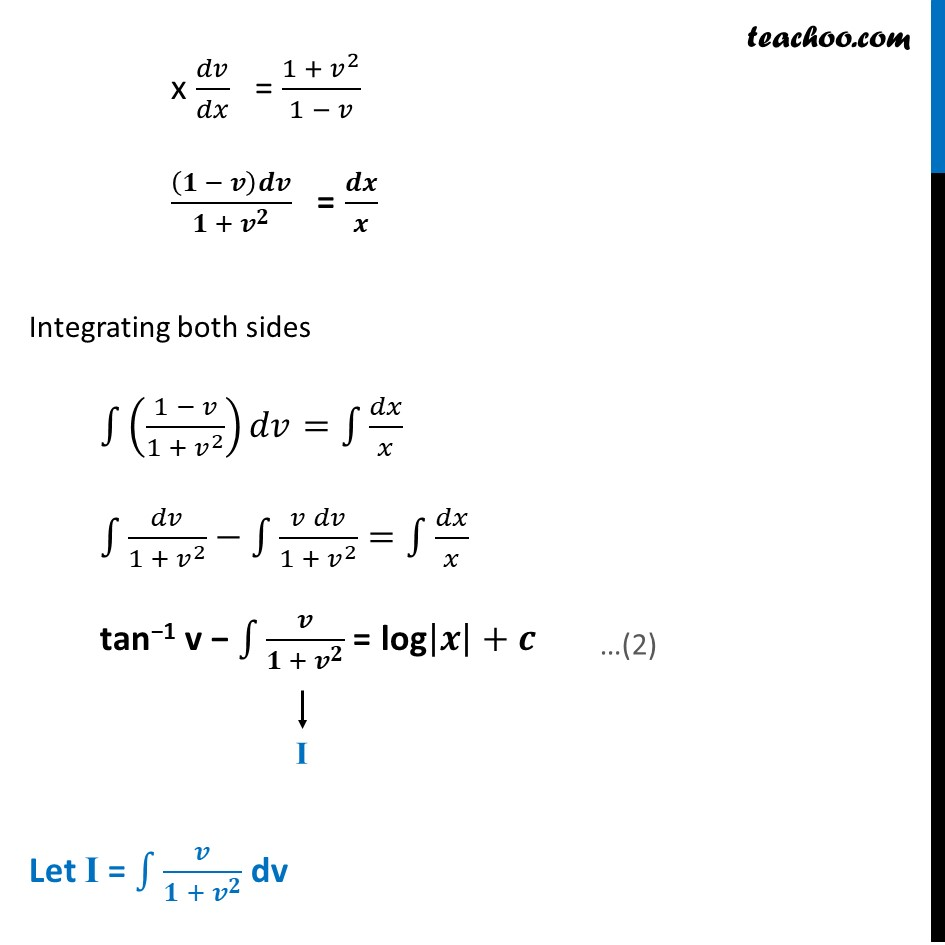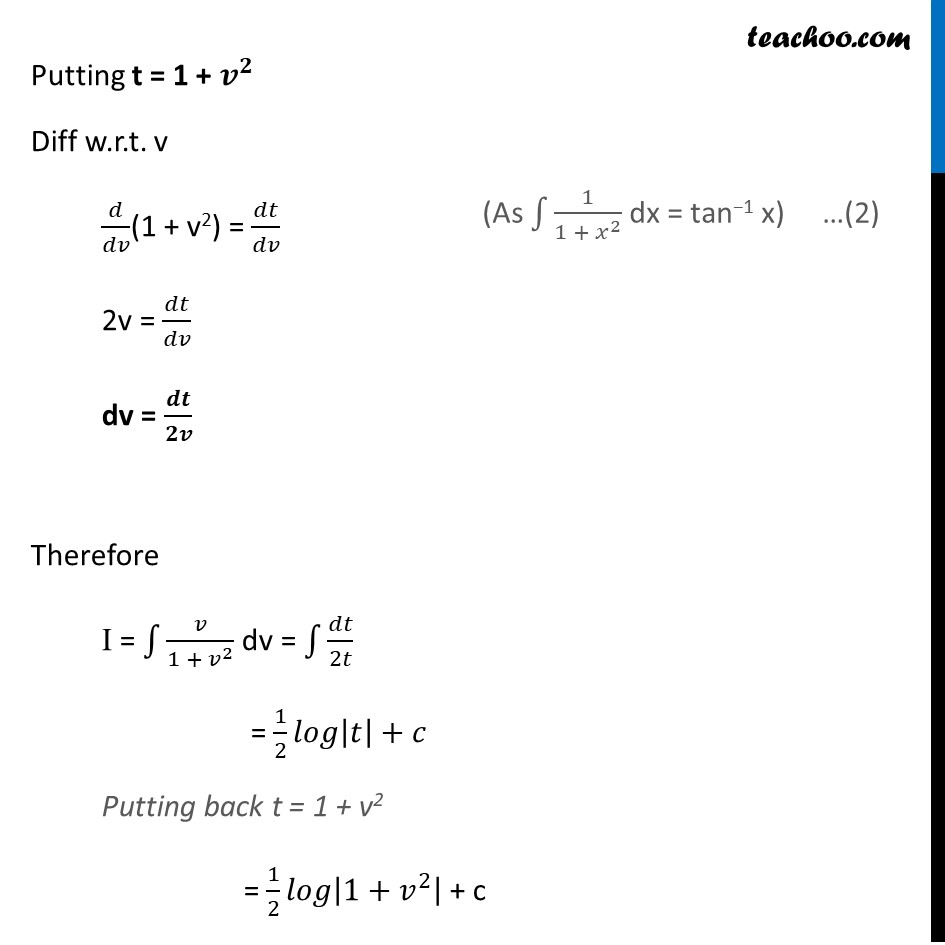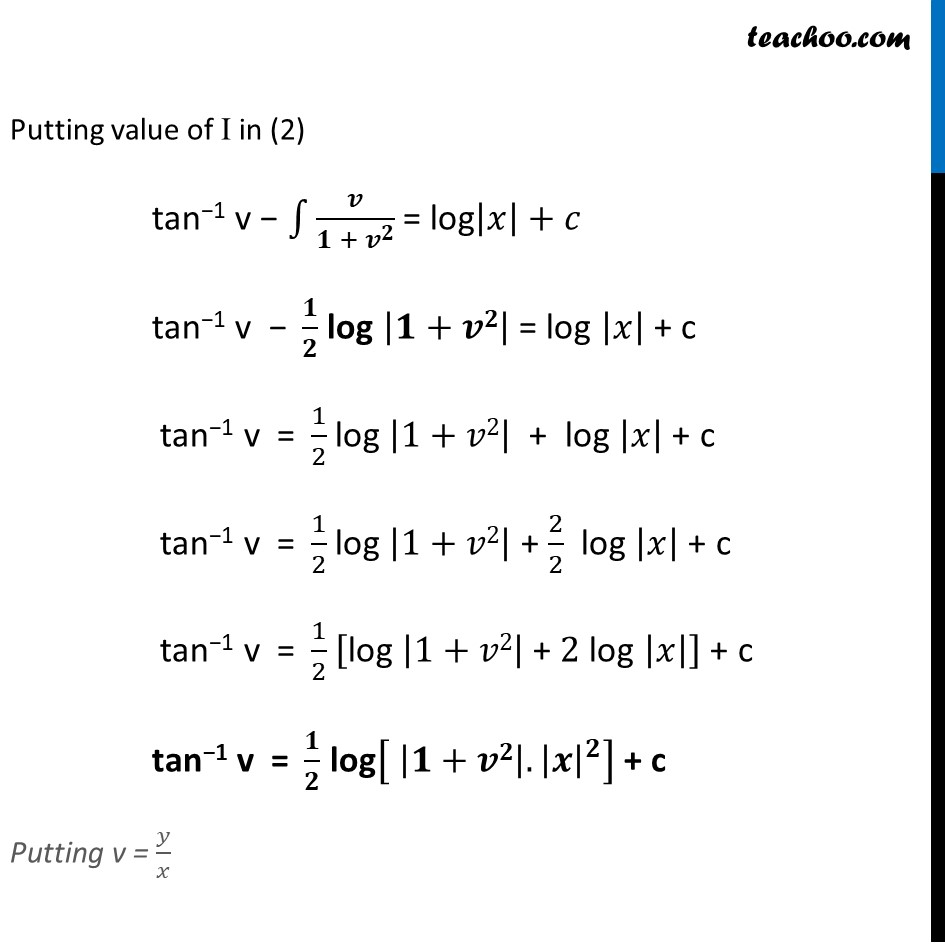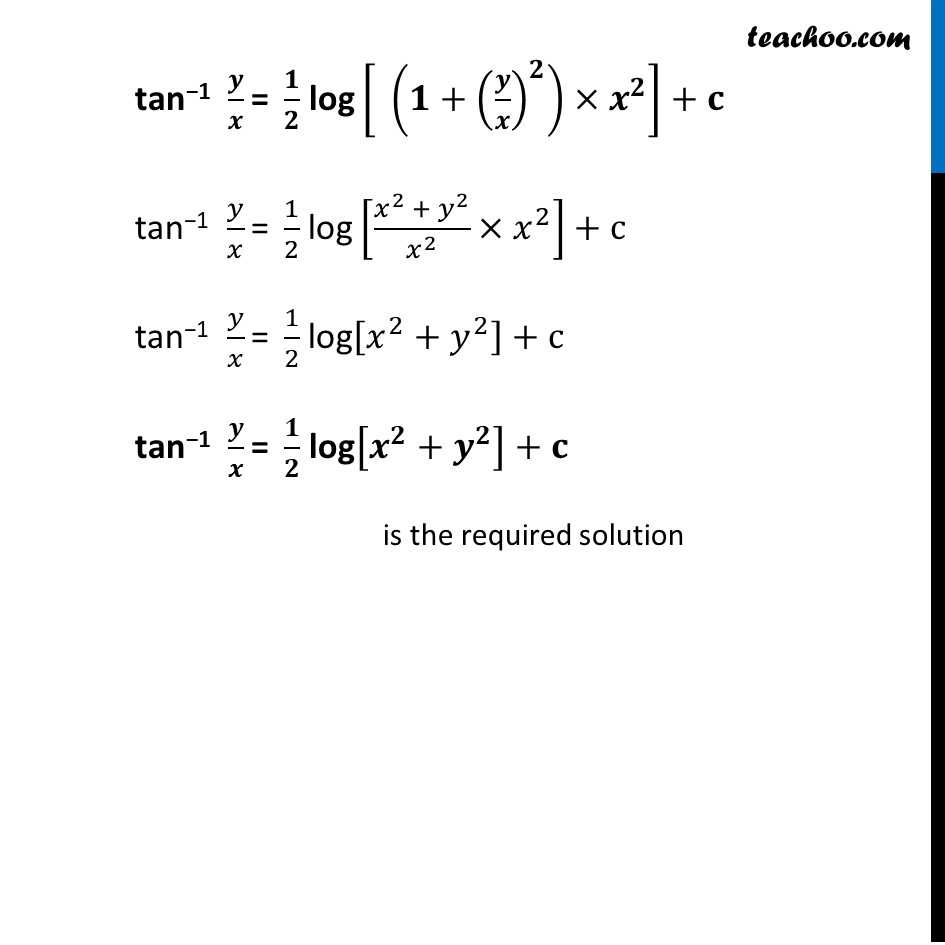Learn in your speed, with individual attention - Teachoo Maths 1-on-1 Class

### Transcript

Ex 9.4, 3 In each of the Exercise 1 to 10, show that the given differential equation is homogeneous and solve each of them. (x−y)𝑑y−(x+y)𝑑𝑥=0 Step 1: Find 𝑑𝑦/𝑑𝑥 (x − y) dy − (x + y) dx = 0 (x − y) dy = (x + y) dx 𝒅𝒚/𝒅𝒙 = (𝒙 + 𝒚)/(𝒙 − 𝒚) Step 2: Put 𝑑𝑦/𝑑𝑥 = F(x, y) and find out F(𝜆x, 𝜆y) F(x, y) = (𝑥 + 𝑦)/(𝑥 − 𝑦) F(𝜆x, 𝜆y) = (𝜆𝑥 + 𝜆𝑦)/(𝜆𝑥−𝜆𝑦) = (𝜆(𝑥 + 𝑦))/(𝜆 (𝑥 − 𝑦)) = (𝑥 + 𝑦)/(𝑥 − 𝑦) = F(x, y) ∴ F(𝜆x, 𝜆y) = 𝜆0 F(x, y) Hence, F(x, y) is a homogenous Function of with degree zero So, 𝑑𝑦/𝑑𝑥 is a homogenous differential equation. Step 3: Solving 𝑑𝑦/𝑑𝑥 by putting y = vx Putting y = vx. Differentiating w.r.t. x 𝑑𝑦/𝑑𝑥 = x 𝑑𝑣/𝑑𝑥+𝑣𝑑𝑥/𝑑𝑥 𝒅𝒚/𝒅𝒙 = 𝒙 𝒅𝒗/𝒅𝒙 + v Putting value of 𝑑𝑦/𝑑𝑥 and y = vx in (1) 𝑑𝑦/𝑑𝑥 = (𝑥 + 𝑦)/(𝑥 − 𝑦) x 𝒅𝒗/𝒅𝒙 + v = (𝒙 + 𝒙𝒗)/(𝒙 − 𝒙𝒗) x 𝑑𝑣/𝑑𝑥 + v = (𝑥 (1 + 𝑣))/(𝑥(1 − 𝑣)) x 𝑑𝑣/𝑑𝑥 + v = ((1 + 𝑣))/((1 − 𝑣)) x 𝑑𝑣/𝑑𝑥 = ((1 + 𝑣))/((1 − 𝑣)) − v x 𝑑𝑣/𝑑𝑥 = (1 + 𝑣 − 𝑣(1 − 𝑣))/(1 − 𝑣) x 𝑑𝑣/𝑑𝑥 = (1 + 𝑣 − 𝑣 + 𝑣^2)/(1 − 𝑣) x 𝑑𝑣/𝑑𝑥 = (1 + 𝑣^2)/(1 − 𝑣) (𝟏 − 𝒗)𝒅𝒗/(𝟏 + 𝒗^𝟐 ) = 𝒅𝒙/𝒙 Integrating both sides ∫1▒((1 − 𝑣)/(1 + 𝑣^2 )) 𝑑𝑣=∫1▒𝑑𝑥/𝑥 ∫1▒𝑑𝑣/(1 + 𝑣^2 )−∫1▒(𝑣 𝑑𝑣)/(1 + 𝑣^2 )=∫1▒𝑑𝑥/𝑥 tan−1 v − ∫1▒𝒗/(𝟏 + 𝒗^𝟐 ) = log|𝒙|+𝒄 Let I = ∫1▒𝒗/(𝟏 + 𝒗^𝟐 ) dv Putting t = 1 + 𝒗^𝟐 Diff w.r.t. v 𝑑/𝑑𝑣(1 + v2) = 𝑑𝑡/𝑑𝑣 2v = 𝑑𝑡/𝑑𝑣 dv = 𝒅𝒕/𝟐𝒗 Therefore I = ∫1▒𝑣/(1 + 𝑣^2 ) dv = ∫1▒𝑑𝑡/2𝑡 = 1/2 𝑙𝑜𝑔|𝑡|+𝑐 Putting back t = 1 + v2 = 1/2 𝑙𝑜𝑔|1+𝑣^2 | + c (As ∫1▒1/(1 + 𝑥^2 ) dx = tan−1 x) Putting value of I in (2) tan−1 v − ∫1▒𝒗/(𝟏 + 𝒗^𝟐 ) = log|𝑥|+𝑐 tan−1 v "− " 𝟏/𝟐 log |𝟏+𝒗𝟐| = log |𝑥| + c tan−1 v "= " 1/2 log |1+𝑣2| + log |𝑥| + c tan−1 v "= " 1/2 log |1+𝑣2| + 2/2 log |𝑥| + c tan−1 v "= " 1/2 ["log " |1+𝑣2|" + " 2" log " |𝑥|] + c tan−1 v "= " 𝟏/𝟐 "log" [" " |𝟏+𝒗𝟐|.|𝒙|^𝟐 ] + c Putting v = 𝑦/𝑥 tan−1 𝒚/𝒙 "= " 𝟏/𝟐 "log" [" " (𝟏+(𝒚/𝒙)^𝟐 )×𝒙^𝟐 ]+𝐜 tan−1 𝑦/𝑥 "= " 1/2 "log" [(𝑥^2 + 𝑦^2)/𝑥^2 ×𝑥^2 ]+c tan−1 𝑦/𝑥 "= " 1/2 "log" [𝑥^2+𝑦^2 ]+c tan−1 𝒚/𝒙 "= " 𝟏/𝟐 "log" [𝒙^𝟐+𝒚^𝟐 ]+𝐜 is the required solution